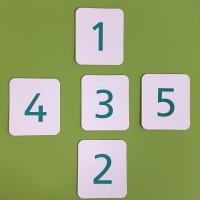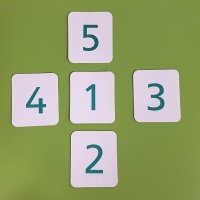#### You may also like### Counting Factors

Is there an efficient way to work out how many factors a large number has?### Summing Consecutive Numbers

15 = 7 + 8 and 10 = 1 + 2 + 3 + 4. Can you say which numbers can be expressed as the sum of two or more consecutive integers?### Oh! Hidden Inside?

Find the number which has 8 divisors, such that the product of the divisors is 331776.

# Magic Crosses

##### Age 7 to 14 Challenge Level:
This problem was featured in the NRICH Webinar on December 12th 2018. Visit the webinar page to watch the video.

### Why do this problem?

This low threshold high ceiling problem gives students opportunities to perceive structure in a simple case, make generalisations based on that structure, and go on to pose their own questions.

By choosing to represent the underlying patterns in different ways, such as Numicon, digit cards, the interactive Magic Cross Tester, or even algebra, we hope students will be able to persevere and find convincing arguments and proofs to explain the patterns they notice.

### Possible approach

Show students the two crosses below:"What do you notice about the two crosses? What's the same? What's different?"
Possible responses:
They both use the digits from 1 to 5
They both have a 4 on the left
They are the same shape
The one on the left has a horizontal total of 12 but the one on the right has a horizontal total of 8
The one on the right has an equal horizontal and vertical total

Establish that the cross on the right is a magic cross because the horizontal and vertical totals are the same.

"I wonder how many magic crosses there are, using the digits 1, 2, 3, 4 and 5."
Give students some time to work on the problem. Some students might ask whether it counts as a different cross if the horizontal and vertical lines are swapped, or if the left and right digits are swapped, for example. This is a good opportunity to discuss symmetry and commutativity, and to suggest that mathematicians would probably want to identify families of crosses rather than each individual cross within a family.

After students have had time to explore, bring them together to share the different crosses they have found, and pose the question "How can we be sure we've found them all?"

Here are some explanations students might come up with in order to convince themselves that there are only three magic crosses using the digits 1, 2, 3, 4 and 5:
• As the numbers from 1 to 5 add up to an odd total, the number in the middle must be odd.
• If we put a 2 or a 4 at the centre, we'd have an odd total left over to split between the two lines, so it can't be done equally.
• If there was an even number at the centre, there'd be three odds and one even left, so they can't be split to make the same total.
Next, invite the class to think of other questions they could explore. Here are some suggestions:

Are there any magic crosses that use the numbers 2, 3, 4, 5, 6?
Or 3, 4, 5, 6, 7?
Or 98, 99, 100, 101, 102?

How many magic crosses can you find with the number 50 in the middle?

What if the numbers went up in 2s instead?
Or 3s?
Or 10s, or 20s, or 100s?

Give students some time to work on the chosen questions, and then bring the class together to share any findings, convincing arguments and proofs.

Alternatively, you could set a challenge:
"In a while, I am going to choose a number bigger than 100, and challenge you to create a magic cross which contains my chosen number. You need to develop a strategy so that you can do this for any number that I choose!"

Then finish the lesson by challenging each pair of students to show that their strategy works!

### Key questions

How do you know that is/is not a magic cross?
How will you keep track of what you have found so far?
How will you know when you have found all the possible solutions?

### Possible extension

The task Magic Letters starts off with Vs which are structurally the same as the crosses in this task, but invites students to consider Magic Ls, Ns and Ws.

### Possible support

Giving students digit cards or inviting them to write the numbers on small pieces of paper that can be moved around easily might help them to experiment with different arrangements.